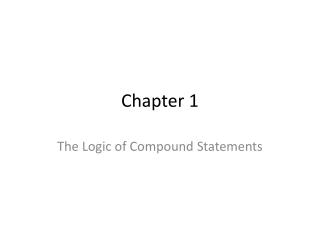Download PresentationChapter 1

# Chapter 1 - PowerPoint PPT PresentationDownload Presentation## Chapter 1

- - - - - - - - - - - - - - - - - - - - - - - - - - - E N D - - - - - - - - - - - - - - - - - - - - - - - - - - -
##### Presentation Transcript

1. Chapter 1 The Logic of Compound Statements

2. Section1.3 Valid & Invalid Arguments

3. Review • Review of last lecture • Conditional Statement • if-then, -> • p -> q ~pvq • Negation of Conditional • ~(p -> q) p^ ~q • Contrapositive of Conditional • p -> q ~q -> ~p • Review • Converse of Conditional • (p->q) is (q->p) • Inverse of Conditional • (p->q) is (~p->q) • Converse  Inverse • Biconditional • “p if, and only if q”, p <-> q, TRUE when both p and q have same logic value

4. Testing Argument Form • Identify the premises and conclusion of the argument form. • Construct a truth table showing the truth values of all the premises and the conclusion. • If the truth table reveals all TRUE premises and a FALSE conclusion, then the argument form is invalid. Otherwise, when all premises are TRUE and the conclusion is TRUE, then the argument is valid.

5. Example • If Socrates is a man, then Socrates is mortal. • Socrates is a man. • :. Socrates is mortal. • Syllogism is an argument form with two premises and a conclusion. Example Modus Ponens form: • If p then q. • p • :. q

6. Example Valid Form • pv (qvr) • ~r • :. pvq

7. Example Invalid Form • p -> qv ~r • q -> p ^ r • :. p -> r

8. Modus Tollens • If p then q. • ~q • :. ~p • Proves it case with “proof by contradiction” • Example: • if Zeus is human, then Zeus is mortal. • Zeus is not mortal. • :. Zeus is not human.

9. Examples • Modus Ponens • “If you have a current password, then you can log on to the network” • “You have a current password” • :. ??? • Modus Tollens • Construct the valid argument using modus tollens. • p->q, ~q, :. ~p • What is p and q? • What is ~q?

10. Rules of Inference • Rule of inference is a form of argument that is valid. • Modus Ponens, Modus Tollens • Generalization, Specialization, Elimination, Transitivity, Proof by Division, etc.

11. Rules of Inference • Generalization • p :. pvq • q :. pvq • Specialization • p ^ q :. p • p ^ q :. q • Example: • Karl knows how to build a computer and Karl knows how to program a computer • :. Karl knows how to program a computer

12. Rules of Inference • Elimination • pvq, ~q, :. p • pvq, ~q, :. p • Example • Karl is tall or Karl is smart. • Karl is not tall. • :. Karl is smart. • x-3=0 or x+2=0 • x ~< 0 • :. x = 3 (x-3=0)

13. Rules of Inference • Transitivity (Chain Rule) • p -> q, q -> r, :. p -> r • Example • If 18,486 is divisible by 18, then 18486 is divisible by 9. • If 18,486 is divisible by 9, then the sum of the digits of 18,486 is divisible by 9. • :. 18,486 is divisible by 18, then the sum of the digits 18,486 is divisible by 9.

14. Rules of Inference • Proof by Division • pvq, p->r, q->r, :.r • Example • x is positive or x is negative. • If x is positive, then x2 > 0. • If x is negative, then x2 > 0. • :. x2 > 0

15. Fallacies • A fallacy is an error in reasoning that results in an invalid argument. • Converse Error • If Zeke is a cheater, then Zeke sits in the back row. • Zeke sits in the back row. • :. Zeke is a cheater. • Inverse Error • If interest rates are going up, then stock market prices will go down. • Interest rates are not going up. • :. Stock market prices will not go down.

16. Contradictions and Valid Arguments • Contradiction Rule – If you can show that the supposition that statement p is false leads logically to a contradiction, then you can conclude that p is true. • ~p -> c, :. p